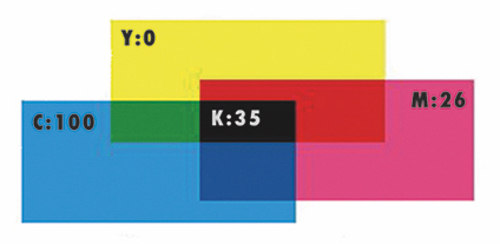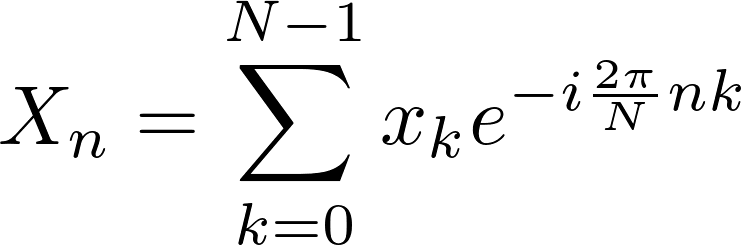# The Math behind the Music:

## CrimsonRobot | w4rner| pmj714

For musicians like one of data vis specialists cerulean, sounds are a wonderful tool, which can be combined and crafted to form pieces of music. But we often take for granted the science behind the sounds. Let's explore!

Sound can be thought of as oscillations in air density over time. Such oscillations can be described by waves which have the following two parameters: 1). Frequency: the number of oscillations occurring in one second. 2). Amplitude: the height of the wave. In music, different notes correspond to different frequencies, and loudness corresponds to amplitude. Explore the effect of changing these two parameters on this wave!

When two waves intersect in space, their values add linearly. Create two waves traveling towards each other and watch them combine when they meet!

Let's consider a chord in music. It is the addition of many notes, and therefore many sounds. Build your own 'musical chord' 'by selecting combinations of the following 6 waves!

Now let's imagine cerulean recorded a song and wants a Bass Boost. In terms of waves, this means raising the amplitude of the lowest frequency waves (in this case, the 4th from the left has a frequency of 0.5Hz). But how can they figure out from a combined wave like the one shown, what the individual waves forming it are like?

cerulean's mathematical magicians Jack & Patrick to the rescue! They know some math - specifically the Fourier Transform. Fear not cerulean: this it the only formula you'll have to see!The exponential term is known as Euler's formula, which relates to the Unit Circle. To explore how a wave is related to the Unit Circle, we have included an example of a sine wave, which plots the y co-ordinate as you move around the Unit Circle. Move the point along the wave using the slider!

Now back to the problem! You'll notice the following wave is the combination formed earlier when all 6 waves were added together. Let's decompose this wave using a Fourier Transform: by clicking the white square!
Fourier Transform #N Samples

Where there are peaks in the dots, this identifies the Frequency of the component waves. Check out the bass frequencies to the left! You can also play with the slider to see how the chosen Number of Samples affects the Transform.

Know a little math and want to dig deeper? Here's a starter: Euler's formula exhibits a similar periodicity when mapped over time with the rate it travels around the circle given by f. We then have that, over a period of time, the product of the wave function mapped onto a circle and of Euler's formula is greatest when the two make the same number of rotations about the circle over a period of time, i.e. when they have the same frequency. It also happens that when the two are out of sync, their product is relatively small. Below we can see what happens when we sample a period of time and calculate the Fourier transform of the complicated sum of waves we had above. For our sum of six waves, we have six distinct peaks! The axis of the transform is not directly in terms of frequency, but rather in terms of the period times the frequency, Tk. One simply needs to divide by our period of 200*pi to determine the frequency. Further, the number of frequencies the Fourier Transform can detect is limited to the half the number of samples we take.

Now we know a little math behind the music, let's get back to the studio to record some new ceruleansounds! Math Rock anyone?